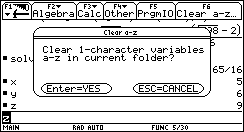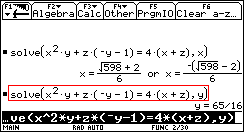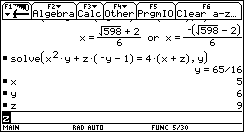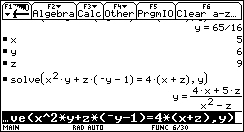IDEA: clear a-z cmd - Printable Version +- HP Forums (https://www.hpmuseum.org/forum) +-- Forum: HP Calculators (and very old HP Computers) (/forum-3.html) +--- Forum: HP Prime (/forum-5.html) +--- Thread: IDEA: clear a-z cmd (/thread-7990.html) IDEA: clear a-z cmd - compsystems - 03-21-2017 03:01 PM Hello sorry for my bad english One of the problems for students, when a calculator as HP-PRIME or TI-89 stores a-z user variables, is that if one does not delete the variables, the results are evaluated, without showing the general or symbolic solutions. See publication in the following link http://courses.wccnet.edu/~palay/math179/intslv92.htm The TI68k have an option to remove the symbolic variables a-z, I think it would be a good option to incorporate it into the HP-PRIME for cas MODE, The RESTART CMD works but deletes all definitions =(, then a single command is required for the alphabetic variables, or expand RESTART CMD, accepting arguments RESTART([arg]) RESTART( "ALL" ); RESTART( "a-z" );CAS VIEW PHP Code: `CAS VIEWCASE 1:  Preassigning values for x,y,z lowercasex:=5; y:=6; z:=9;//1.1: solve lowercase & Maximum simplifysolve( x^2*y+z*(-y-1) = 4*(x+z), x ); // returns { (-√(598)+2)/6,  (√(598)+2)/6 }  // OK, As x,y,z have values, solve cmd solve then evaluateapprox(Ans); {  −3.742 , 4.409 }  // -> x=4.40 or x=−3.74 // ok//1.2: SOLVE uppercaseSOLVE( x^2*y+z*(-y-1) = 4*(x+z), x );  orSOLVE( x^2*y+z*(-y-1) = 4*(x+z), x, 6 );// returns 4.40900642021 SOLVE( x^2*y+z*(-y-1) = 4*(x+z), x, -6 );// returns −3.742 CASE 2: Deleting variables x,y,zpurge(x,y,z); //2.1: solve lowercasesolve( x^2*y+z*(-y-1) = 4*(x+z), x );  // returns { (√(y^2*z+5*y*z+4)+2)/y,   (-√(y^2*z+5*y*z+4)+2)/y } // Symbolic result for X1/2{ (√(y^2*z+5*y*z+4)+2)/y, (-(√(y^2*z+5*y*z+4))+2)/y}|({y = 6,z = 9}); returns{  4.409, −3.742  }solve( x^2*y+z*(-y-1) = 4*(x+z), y );  // returns {(4*x+5*z)/(x^2-z)}// Symbolic result for Y{(4*x+5*z)/(x^2-z)}|({x = 5,z = 9}); returns {65/16}//2.2: SOLVE uppercaseSOLVE( x^2*y+z*(-y-1) = 4*(x+z), x, 6 );  returns "Error: Bad Guess(es)" SOLVE( x^2*y+z*(-y-1) = 4*(x+z), x, -6 ); returns "Error: Bad Guess(es)"SOLVE( x^2*y+z*(-y-1) = 4*(x+z), y, -6 ); returns "Error: Bad Guess(es)"CASE 3:  Non-zero values for X uppercaseX:=5; Y:=6; Z:=9;//3.1: solve lowercase  solve( X^2*Y+Z*(-Y-1) = 4*(X+Z), X); // returns { (-√(598)+2)/6,  (√(598)+2)/6 }  // OK, As x,y,z have values, solve cmd solve then evaluateapprox(Ans); {  −3.742 , 4.409 }  // -> x=4.40 or x=−3.74 // ok//3.2: SOLVE uppercaseSOLVE( X^2*Y+Z*(-Y-1) = 4*(X+Z), X);  orSOLVE( X^2*Y+Z*(-Y-1) = 4*(X+Z), X, 6); // returns 4.40900642021  SOLVE( X^2*Y+Z*(-Y-1) = 4*(X+Z), X, -6);// returns −3.742  CASE 4: Restting variables x,y,zX:=0; Y:=0; Z:=0; //4.1: solve lowercasesolve( X^2*Y+Z*(-Y-1) = 4*(X+Z), X); // returns { 0 }//4.2: SOLVE uppercaseSOLVE( X^2*Y+Z*(-Y-1) = 4*(X+Z), X, 6);  returns0 ` HOME VIEW PHP Code: `HOME VIEWSOLVE(Eq, var, guess) // SOLVE CMD for HOMECASE 5:X:=5; Y:=6; Z:=9;SOLVE( X^2*Y+Z*(-Y-1) = 4*(X+Z), X); returns 4.40900642021 solve( X^2*Y+Z*(-Y-1) = 4*(X+Z), X);  returns {X}CASE 6: Restting variables x, y, zX:=0; Y:=0; Z:=0; SOLVE( X^2*Y+Z*(-Y-1) = 4*(X+Z), X); returns 4.40900642021solve( X^2*Y+Z*(-Y-1) = 4*(X+Z), X); returns {X} ` RE: IDEA: clear a-z cmd - Arno K - 03-21-2017 08:33 PM Is √(y^2*z+5*y*z+4)+2)/y different from √(598)+2)/6) with y=6 and z=9? No, so the result is correct. So one has to be aware of the variables one has used before and then gets the desired symbolic results. I myself usually nearly never assign values to variables used in cas and have some rules I follow when I do so. Nevertheless the idea of spending purge some more parameters is not bad. Arno RE: IDEA: clear a-z cmd - cyrille de brébisson - 03-22-2017 05:48 AM Hello, One of the issue here is the meaning of the word "variable". In symbolic math, a variable is a name used in replacement of an unknown value. In the symbolic word, a variable does not have any value (at least most of the time). In lower grade math, in "computational" math, in physics or in programming worlds, a variable is a named container for a value. a Variable always has a value which it temporary replaces for clarity, readability or to allow a series of final computations with various values for the said variables. Prime does have both these worlds in it. Home is definitely computational math. Cas is symbolic. But the 2 also interact to add to the confusionThe end result is Prime. Power and flexibility, but at the cost of the need to understand such subtility. In your case, remember that by default, A-Z variables always have a value (computational) and a-z (lowercase) do not (symbolic). And you should be OK. Hope this helps. Cyrille RE: IDEA: clear a-z cmd - KeithB - 03-22-2017 03:03 PM That is fine for FORTRAN programmers that can use I for a loop variable, but what about us C programmers that have to use i? 8^) I suggest that the OP write his own darn function/program if it is that important to him. RE: IDEA: clear a-z cmd - compsystems - 03-22-2017 06:42 PM The SOLVE in capital letters is not documented in the CATALOG HELP Quote:solve Returns a list of the solutions (real and complex) to a polynomial equation or a set of polynomial equations. solve(Eq,[Var]) or solve({Eq1, Eq2,…}, [Var]) or solve(Eq, Var=Guess) or solve(Eq, Var=Val1…Val2) Quote:SOLVE Solves an equation for one of its variables. Solves the equation En for the variable var, using the value of guess as the initial value for the value of the variable var. If En is an expression, then the value of the variable var that makes the expression equal to zero is returned. SOLVE(En,var,guess) This function also returns an integer that is indicative of the type of solution found, as follows: 0—an exact solution was found 1—an approximate solution was found 2—an extremum was found that is as close to a solution as possible 3—neither a solution, an approximation, nor an extremum was found I do not understand the last part [0 - 3] Update the examples of the first post, I think that THE DIFFERENT CASES should have a more detailed explanation by Bernard RE: IDEA: clear a-z cmd - parisse - 03-23-2017 04:52 PM solve(equation) solves equation with respect to x solve(equation,var) solves equation with respect to var solve([eq1,eq2,..],[var1,var2,...]) solve polynomial system with respect to var1,var2,... The last syntaxes call the numeric solver, using an iterative method (var=guess) or bisection (var=a..b). RE: IDEA: clear a-z cmd - compsystems - 03-24-2017 08:21 PM SOLVE CMD in capitals should not evaluate after solving, when the variables are also capitals, because by default X, Y, ... are initialized to zero and there is no way to eliminate previously. Please check the examples above Thank you